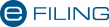# FAQ: Why does the time-apportionment method give different results in the scenarios set out below?

SCENARIO 1:
An individual acquired an office block 16 years before 1 October 2001 for R250 000. A set of storerooms were added at a cost of R60 000 two years before 1 October 2001. A new wing was added at a cost of R300 000, a year after 1 October 2001. The office block was sold for R2 000 000, two years after 1 October 2001.
SCENARIO 2:
The same facts but the cost of adding the storeroom and new wing was all incurred one year before 1 October 2001. Why is it that if you incurred it over a period your gain is higher than if you incurred it once?
SCENARIO 1:
Expenditure incurred pre- and post- valuation date
Pre-valuation date expenditure is R250 000 + R60 000 = R310 000
Post-valuation date expenditure = R300 000
Total expenditure = R610 000
Proceeds R2 000 000
Period property held before valuation date = 16 years.
Period held after valuation date = 2 years.
Total = 18 years.
Pre-valuation date proceeds = Proceeds x pre-valuation date expenditure
Total expenditure = R2 000 000 x R310 000
R610 000
= R1 016 393
Gain produced by pre-valuation date expenditure =R1 016 393 – R310 000 = R706 393
Portion of capital gain attributable to period before valuation date = R706 393 x 16 years
18 years
= R627 905
Valuation date value = R310 000(pre-valuation date expenditure) R627 905 = R937 905
Total base cost = R937 905 R300 000 = R1 237 905
Capital gain = proceeds – base cost = R2 000 000 – R1 237 905
= R762 095
SCENARIO 2:
Expenditure all incurred in the year of purchase before CGT
Proceeds are all attributable to pre-valuation date expenditure = R2 000 000
Total costs = R610 000
Gain = R1 390 000
Time apportionment base costR1 3900 000 x 16/18years = R1 235 556
Base cost = R610 000 R1 235 556 = R1 845 556
Capital gain =R2 000 000 – R1 845 556 = R154 444
It is not the fact that you incurred the costs over a period rather than all at once that necessarily affects the result. The gain in scenario 1 is higher than in scenario 2 because nearly half the costs were incurred after valuation date and thus generated nearly half of the post CGT gain.
In scenario 2 all these post-valuation date costs are thrown back 16 years to the date of acquisition prior to the valuation date with the result that 16/18 of the gain is attributable to the pre-valuation date period.

Last Updated: